## ICSE Class 9 Physics Sample Question Paper 1 with Answers

[Max Marks : 80
[2 Hours]

General Instructions

• Answers to this Paper must be written on the paper provided separately.
• You will not be allowed to write during the first 15 minutes.
• This time is to be spent in reading the question paper.
• The time given at the head of this Paper is the time allowed for writing the answers.
• Section I is compulsory. Attempt any four questions from Section II.
• The intended marks for questions or parts of questions are given in brackets [ ].

Section – I
(Attempt all questions from this section) [40 Marks]

Question 1.
(a) (i) Define the least count of an instrument. 
(ii) What is the Least count of a standard laboratory micrometer screw gauge?
(b) A spring balance is used to find the weight of a body X on the surface of the moon. The mass of the body X is 2 kg and its weight is recorded as 3.4 N. The weight of another
body Y recorded by the same balance is found to be 7.65 N. Calculate the mass of the body Y. 
(c) State two differences between mass and weight. 
(d) Two pendulums P and Q have equal lengths but their bobs weigh 10 gf and 20 gf respectively. 
(i) Compare their time periods.
(e) Why do we need to run a certain distance before getting into a moving bus? 
(a) (i) The least count of an instrument is the smallest measurement that can be taken accurately with it.
(ii) The least count of a standard laboratory micrometer screw gauge is 0.0001 cm.

(b)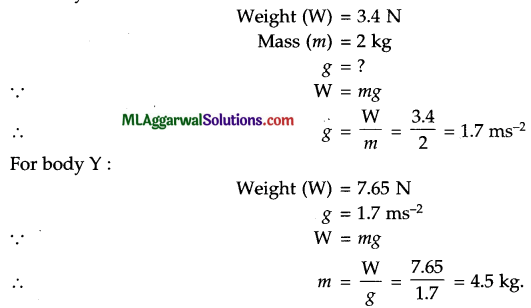(c)

 Mass Weight Mass is a scalar quantity. Weight is a vector quantity. Its S.I. unit is kilogram (kg) Its S.I. unit is newton (N).

(d) (i) 1:1.
(ii) Time period does not depend upon the weight of the bob.(e) The reason is that by running, we bring our body in the state of motion. When the body is in motion, it is easier to get into a moving bus.

Question 2.
(a) Copy the diagram below and clearly mark the directions of the forces that act on it and name the forces.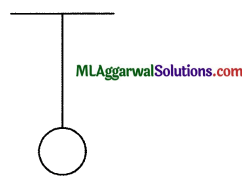(b) If I travel from Mumbai to Pune (150 km) in 2 1/2 hrs via the Express Highway and return to Mumbai via the old Highway (180 km) in 3 1/2 hrs, calculate the average velocity during the entire journey. 
(c) Define retardation and give an example of a body having this motion. 
(d) Copy the diagram and complete the path of the rays A and B.(e) State the purpose of using the following in an electric circuit.  
(i) Ammeter
(ii) Rheostat
(a) The completed diagram is shown alongside. The weight acts downwards and the tension acts upwards.

(b) The average velocity is zero because the displacement is zero.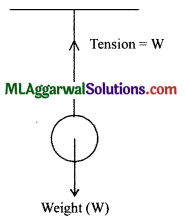(c) Retardation is defined as the decrease in velocity per second.
For example, a train approaching a station must have retardation in order to stop.(d)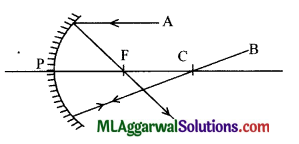Question 3.
(a) A sound wave of frequency 500 Hz and wavelength 0.66 m is travelling in a medium. Calculate the velocity of the wave in the medium.   
(b) Sound waves A and B are travelling in two different media.
Find which wave will be travelling faster, when 
(i) A is travelling in water and B is travelling in CO2.
(ii) A is travelling in CO2 and B is travelling in hydrogen. Also support your answers with reasons.
(c) A body is completely immersed in a fluid. State two factors on which the upthrust acting on the body depends. 
(d) A and B are two metal spheres which are connected with the help of a metal wire. State the direction of flow of electrons in each case.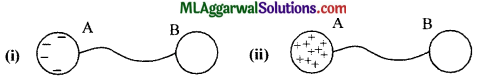(e) Why do we use a convex mirror as a rear view mirror ? 
(a) Given : f = 500 Hz, λ = 0.66 m
We know that, υ = fλ
= 500 x 0.66 330 ms-1

(b) (i) Speed of sound wave in a liquid is greater than the speed in a gas. Hence, wave A travelling in water will travel faster than wave B travelling in carbon dioxide.
(ii) Speed of sound is inversely proportional to density of medium. Hence, wave B travelling in hydrogen will have more speed than wave A travelling in carbon dioxide.

(c) Upthrust on a body due to a fluid depends on the following two factors :
(1) Volume of the body submerged in liquid (or fluid), and
(2) Density of the liquid (or fluid) in which the body is submerged.(d) (i) A to B
(ii) B to A.

(e) A convex mirror diverges the incident light beam and always forms a virtual, small and erect image between its pole and focus. This fact enables the driver to use it as a rear view mirror in vehicles to see all the traffic approaching from behind.

Questions 4.
(a) State two differences between an electromagnet and a permanent magnet. 
(b) Why does a magnet suspended freely from its C.G., always come to rest along the north south direction of the earth ?  
(c) State the second law of thermodynamics in energy flow. (Law of conservation of energy) 
(d) Name and state the principle on which the hydraulic brakes of a car work. 
(e) State two main human activities which are responsible for the increase of carbon dioxide gas in the atmosphere.  

 Electromagnet Permanent Magnet An electromagnet is made of soft iron. A permanent magnet is made of steel. The polarity of an electromagnet can be reversed. The polarity of a permanent magnet can not be reversed.

(b) A magnet suspended freely from its C.G. always comes to rest along the north-south direction of the earth because the north pole of the magnet points towards the geographic north where there is a magnetic south pole attracting it. Similarly, the south pole of the magnet points towards the geographic south where there is a magnetic north pole attracting it.

(c) According to the second law of thermodynamics, when energy is put to work, a part of it is always converted in unuseful form as heat, mainly due to friction and radiation.

(d) The hydraulic brakes of a car work on the principle of hydraulic machine. The principle of each hydraulic machine is that a small force applied on a smaller piston is transmitted to produce a large force on the bigger piston.(e) Two human activities responsible for the increase of carbon dioxide gas are as follows :
(1) The burning of fuels, and
(2) Increase in population.

Section – II
(Attempt any four questions from this section) [40 Marks]

Question 5.
(a) A train is moving at a velocity of 25 ms-1. It is brought to rest by applying the brakes which produces a uniform retardation of 0.5 ms-2. Calculate : 
(i) The velocity of the train after 10s.
(ii) If the mass of the train is 20000 kg then calculate the force required to stop the train.

(b) (i) State the universal law of gravitaiton. 
(ii) Express it in a mathematical form. (Explain the symbols used.)
(iii) State the value of universal gravitational constant in S.I. unit.

(c) Using the following velocity time graph of a body answer the following questions.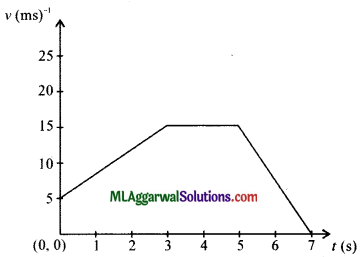(i) During which time intervals is the body moving with variable velocity ?
(ii) What is the acceleration of the body during the interval 3s to 5s ?
(iii) What is the displacement of the body in the last four seconds of its motion ?
(a) (i) Given : u = 25 ms-1, cv= -5 ms-2, t = 10 s
v = u + at
= 25 + (- 0.5) x 10 = 25 – 5 = 20 ms-1

(ii)   m = 20000 kg
F = ma = 20000 x 0.5 = 10000 N.

(b) (i) According to Newton’s universal law of gravitation, the force of attraction acting between the two particles is (1) directly proportional to the product of their masses and (2) inversely proportional to the square of the distance between them.(ii) Let there be two particles of masses m1 and m2 at a separation of r. The magnitude of force of attraction F acting between them is :
$$\mathrm{F}=\mathrm{G} \frac{m_{1} m_{2}}{r^{2}}$$

(iii) The value of gravitational constant G is 6.67 x 10-11 Nm2 kg-2.

(c) (i) From O to 3 s, and 5s to 7 s
(ii) Zero
(iii) Displacement in the last 4 seconds
= Area of trapezium BCDE

Question 6.
(a) (i) Differentiate between the terms supersonic and ultrasonic. 
(ii) State two uses of ultrasound.
(b) State three factors on which the speed of sound depends. 
(c) The speed of sound in air is 320 ms-1 and in water it is 1600 ms-1. It takes 2.5 s for sound to reach a certain distance from the source placed in air.  
(i) Find the distance.
(ii) How much time will it take for sound to travel the same distance when the source is in water ?
(a) (i) Sound of frequency above 20 kHz is called ultrasound or ultrasonic sound whereas supersonic refers to an object which travels with a speed greater than the speed of sound in air.
(ii) Ultrasound is used for making cuts of desired shape in materials like glass. It is also used in surgery to remove cataract.

(b) The speed of sound in a gas is affected by the change in :
(1) Density : Speed of sound is inversely proportional to the square root of density of the gas.
(2) Temperature: The speed of sound in a gas increases with the increase in temperature of the gas.
(3) Humidity : The speed of sound in air increases with the increase in humidity in air.(c) (i) In air : Speed (v) = 320 ms-1
Time (t) =  2.5s
∴ Distance (d) = v x t
= 320 x 2.5 = 800 m

(ii)  In Water : Speed (v) = 1600 ms-1
Distance (d) = 800 m
$$\text { Time }(t)=\frac{d}{v}=\frac{800}{1600}=0.5 \mathrm{s}$$

Question 7.
(a) The diagrams below show pins suspended from the same magnet to their maximum limit in two different cases. State, with a reason, whether the set of pins A or the set of pins B are made out of soft iron. Also define the magnetic process which enables us to suspend the pins one below the other.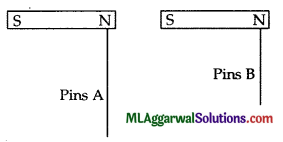(b) (i) Define a secondary cell. 
(ii) Give one example of a secondary cell.
(iii) State one advantage of a secondary cell over a primary cell.

(c) (i) Define a neutral point. 
(ii) In the diagram below AB is a magnet and CD is an iron bar.
Study the diagram and determine the polarities at the ends A, B and D.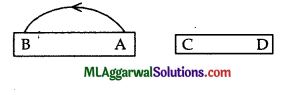(a) Set of pins A are made out of soft iron because the set of pins A have acquired a stronger magnetic field strength as compared to the set of pins B. The process which enables us to suspend the pins is called induced magnetism. The temporary magnetism acquired by a magnetic material when it is kept near (or in contact with) a magnet is called induced magnetism.

(b) (i) A secondary cell is a cell which provides current as a result of chemical reaction which is reversible and so they can be recharged.
(ii) Lead accumulator is an example of a secondary cell.
(iii) A secondary cell can be recharged whereas a primary cell cannot be recharged.(c) (i) Neutral point is a point where the magnetic field of magnet is equal in magnitude to the earth’s horizontal magnetic field, but it is in opposite direction. Thus, the resultant (or net) magnetic field at the neutral points is zero.
(ii) A is north pole, B is south pole and D is north pole.

Question 8.
(a) The area of pistons in hydraulic machine are 6 cm2 and 576 cm2. What force on the smaller piston will support a load of 1152 N on the larger piston ?  
State assumption made in the above calculation.

(b) (i) Define global warming. 
(ii) State two ways by which global warming impacts on life on earth.

(c) Complete the path of the ray AB over plane mirrors M1 and M2 and label all the angles of incidences.(a)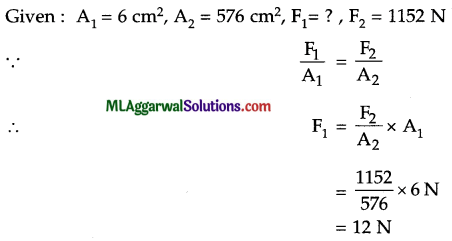Hence, force on smaller piston is 12 N. The assumption is that there is no friction or leakage of liquid

(b) (i) Global warming is the increase in average effective temperature near the earth’s surface due to an increase in the amount of green house gases in its atmosphere
(ii) Due to global warming, there is a change in blooming season of different plants. There is also an increase in heat stroke deaths.(c) The complete ray diagram is as follow :Question 9.
(a) (i) Why does a piece of steel sink in water but float on mercury ? 
(ii) If a bowl is formed from the same steel piece then it can float in water. Why ?

(b) The diagram below shows a frozen pond in a cold region. 
(i) State the expected temperatures at A and B.
(ii) Name the phenomenon responsible for these temperature mentioned in part (i).(c) A metal piece weighs 200 gf in air and 150 gf when completely immersed in water. 
(i) Calculate the relative density of the metal piece.
(ii) How much will it weigh in a liquid of density 0.8 g cm-3?
(a) (i) Mercury has a higher density than steel so it produces a greater upthrust which enables the piece of steel to float on it, but in case of water, the upthrust produced is less than the weight of the steel piece as the density of water is less than that of steel. Hence, it sinks in water.
(ii) When the bowl is formed from the same piece of steel, it can float on water because its volume increases and it will displace a greater volume of water thereby increasing the upthrust.

(b) (i) Temperature at A is 0°C and temperature at B is 4°C.
(ii) The phenomenon responsible for the mentioned temperatures is the anomalous expansion of water.

(c) Given : Weight of metal in air (w1)= 200 gf
Weight of metal in water (w2) = 150 gf
(i) Relative density of metal piece =$$=\frac{w_{1}}{w_{1}-w_{2}}=\frac{200}{200-150}=4$$(ii) Density of liquid = 0.8 g cm-3
Relative density of liquid = 0.8
Let , Weight of metal in liquid = w3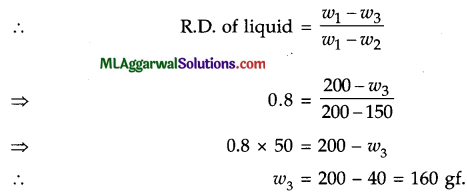Question 10.
(a) Copy and complete the following ray diagram to obtain the image of the object AB kept in front of the concave mirror.(b) An object of height 20 cm is kept at a distance of 48 cm in front of a mirror of focal length 12 cm. If the mirror forms a virtual, diminished image of the object then calculate : 
(i) the distance of the image from the mirror.
(ii) its magnification.(c) Study the diagram below and 
(i) Identify the electrical components labelled A, B and C.
(ii) State whether the circuit given below is open or closed.(a) The complete diagram is as drawn below.(b) Since the image formed is virtual and diminished, the mirror used is convex.
Given : f = 12 cm (positive)
u = 48 cm (negative)
Using the relation,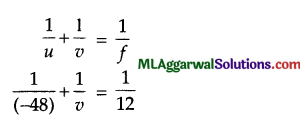Thus, the image is formed at a distance of 9.6 cm behind the mirror.
(ii) Magnification (m) $$=\frac{-v}{u}=\frac{-9.6}{(-48)}=\frac{1}{5}$$
Thus, the size of the image is one-fifth the size of the object.(c) (i) A is the switch, B is the fixed resistance, and C is the cell,
(ii) The given circuit is open.